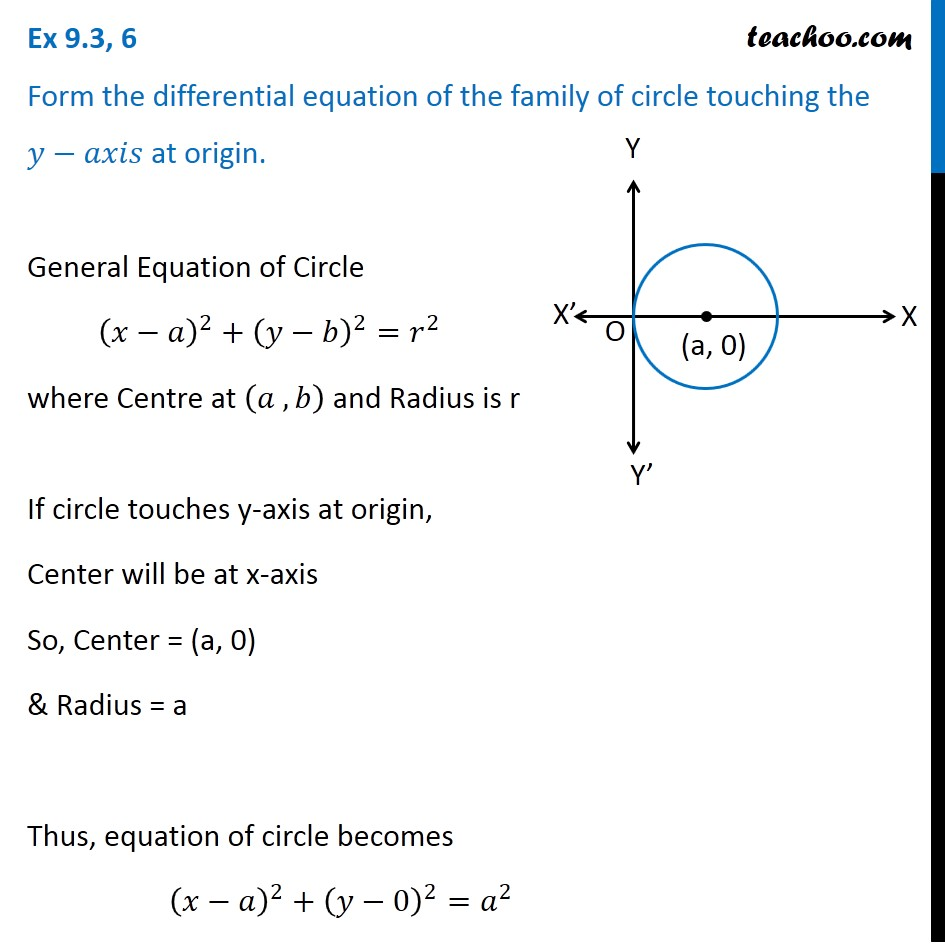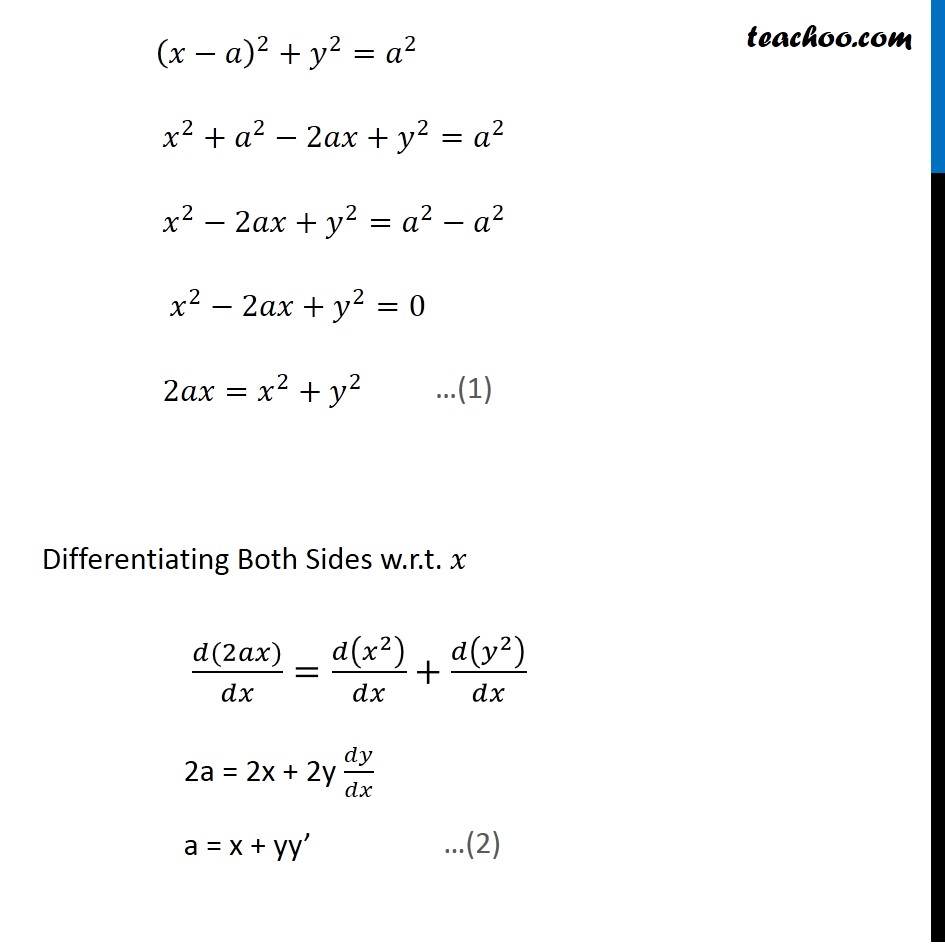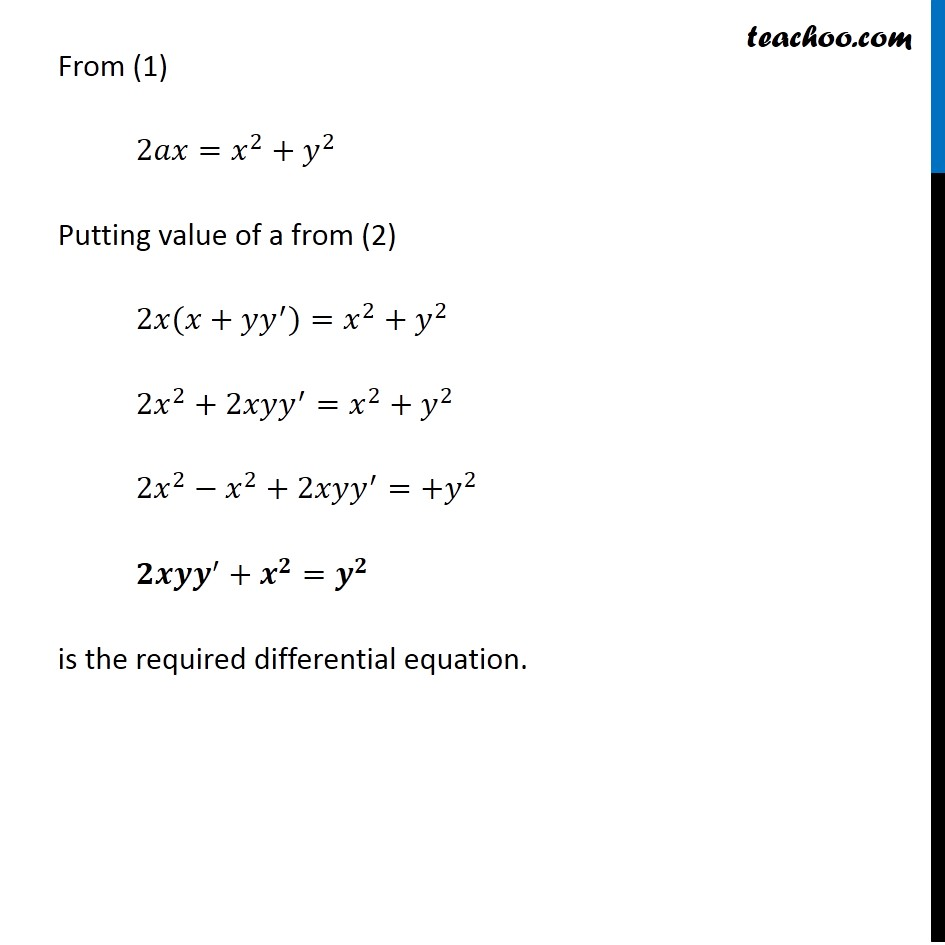Forming Differential equations

Chapter 9 Class 12 Differential Equations
Serial order wiseLearn in your speed, with individual attention - Teachoo Maths 1-on-1 Class

### Transcript

Question 6 Form the differential equation of the family of circle touching the 𝑦−𝑎𝑥𝑖𝑠 at origin. General Equation of Circle (𝑥−𝑎)^2+(𝑦−𝑏)^2=𝑟^2 where Centre at (𝑎 , 𝑏) and Radius is r If circle touches y-axis at origin, Center will be at x-axis So, Center = (a, 0) & Radius = a Thus, equation of circle becomes (𝑥−𝑎)^2+(𝑦−0)^2=𝑎^2 (𝑥−𝑎)^2+𝑦^2=𝑎^2 𝑥^2+𝑎^2−2𝑎𝑥+𝑦^2=𝑎^2 𝑥^2−2𝑎𝑥+𝑦^2=𝑎^2−𝑎^2 𝑥^2−2𝑎𝑥+𝑦^2=0 2𝑎𝑥=𝑥^2+𝑦^2 Differentiating Both Sides w.r.t. 𝑥 (𝑑(2𝑎𝑥))/𝑑𝑥=𝑑(𝑥^2 )/𝑑𝑥+𝑑(𝑦^2 )/𝑑𝑥 2a = 2x + 2y 𝑑𝑦/𝑑𝑥 a = x + yy’ …(1) …(2) From (1) 2𝑎𝑥=𝑥^2+𝑦^2 Putting value of a from (2) 2𝑥(𝑥+𝑦𝑦^′)=𝑥^2+𝑦^2 2𝑥^2+2𝑥𝑦𝑦^′=𝑥^2+𝑦^2 2𝑥^2−𝑥^2+2𝑥𝑦𝑦^′=+𝑦^2 𝟐𝒙𝒚𝒚^′+𝒙^𝟐=𝒚^𝟐 is the required differential equation.